Processing ......FreeComputerBooks.com Links to Free Computer, Mathematics, Technical Books all over the World

Language, Proof and Logic
🌠 Top Free C Programming Books - 100% Free or Open Source
• Title Language, Proof and Logic
• Authors Jon Barwise, John Etchemendy
• Publisher: CSLI Publications; 1st edition (April 1, 2002); 2nd Edition edition (October 15, 2011)
• Paperback: 598 pages
• eBook: PDF Files
• Language: English
• ISBN-10: 157586374X
• ISBN-13: 978-1575863740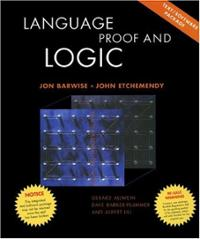Book Description

This textbook covers first-order language in a method appropriate for first and second courses in logic. The unique on-line grading services instantly grades solutions to hundred of computer exercises. It is specially devised to be used by philosophy instructors in a way that is useful to undergraduates of philosophy, computer science, mathematics, and linguistics.

The book is a completely rewritten and much improved version of The Language of First-order Logic. Introductory material is presented in a more systematic and accessible fashion. Advanced chapters include proofs of soundness and completeness for propositional and predicate logic, as well as an accessible sketch of Godel's first incompleteness theorem. The book is appropriate for a wide range of courses, from first logic courses for undergraduates (philosophy, mathematics, and computer science) to a first graduate logic course.

• Jon Barwise (1942-2000) was a mathematician, philosopher and logician. He taught at the Universities of Yale and Wisconsin before becoming the first director of the Center for the Study of Language and Information at Stanford University. From 1990 until his death he was professor of philosophy at Indiana University.
• John Etchemendy has been professor of philosophy at Stanford since 1983. In 2000 he became Provost of Stanford University.
Reviews, Ratings, and Recommendations: Related Book Categories: Read and Download Links:Similar Books:
•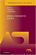Model-Theoretic Logics (Jon Barwise, et al)

This book brings together several directions of work in model theory between the late 1950s and early 1980s. It provides an introduction to the subject as a whole, as well as to the basic theory and examples. Many chapters can be read independently.

•Proof, Sets, and Logic (M. Randall Holmes)

Addressing the importance of constructing and understanding mathematical proofs, this book introduces key concepts from logic and set theory as well as the fundamental definitions of algebra to prepare readers for further study in the field of mathematics.

•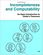Incompleteness and Computability: Gödel's Theorems

This book is an introduction to metamathematics and Gödel's Theorems. It covers recursive function theory, arithmetization of syntax, the first and second incompleteness theorem, models of arithmetic, second-order logic, and the lambda calculus.

•What is Mathematics: Godel's Theorem and Around (K. Podnieks)

This accessible book gives a new, detailed and elementary explanation of the Godel incompleteness theorems and presents the Chaitin results and their relation to the da Costa-Doria results, which are given in full, but with no technicalities.

•Book of Proof, 3rd Edtion (Richard H. Hammack)

This book is an introduction to the language and standard proof methods of mathematics. It lays a foundation for more theoretical courses such as topology, analysis and abstract algebra.

•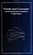Proofs and Concepts: The Fundamentals of Abstract Mathematics

This undergraduate textbook provides an introduction to proofs, logic, sets, functions, and other fundamental topics of abstract mathematics. It helps students transition from solving problems to proving theorems.

•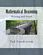Mathematical Reasoning: Writing and Proof (Ted Sundstrom)

Help students to develop logical thinking skills and to think abstractly, and write mathematical proofs using standard methods of mathematical proof including direct proofs, proof by contradiction, mathematical induction, case analysis, counterexamples.

•An Introduction to Mathematical Reasoning (Peter J. Eccles)

The purpose of this book is to introduce the basic ideas of mathematical proof to students embarking on university mathematics. It eases students into the rigors of university mathematics.

Book Categories
 :All CategoriesTop Free BooksRecent BooksMiscellaneous BooksComputer EngineeringComputer LanguagesComputer ScienceData Science/DatabasesJava and Java EE (J2EE)Linux and UnixMathematicsMicrosoft and .NETMobile ComputingNetworking and CommunicationsSoftware EngineeringSpecial TopicsWeb Programming
Other Categories